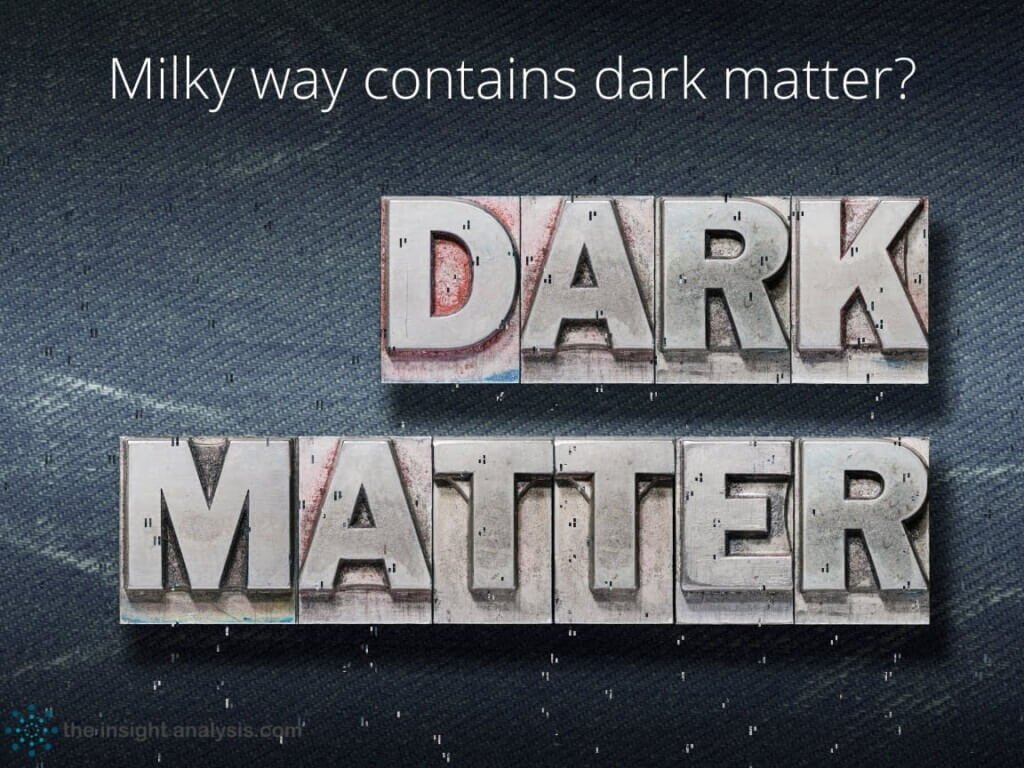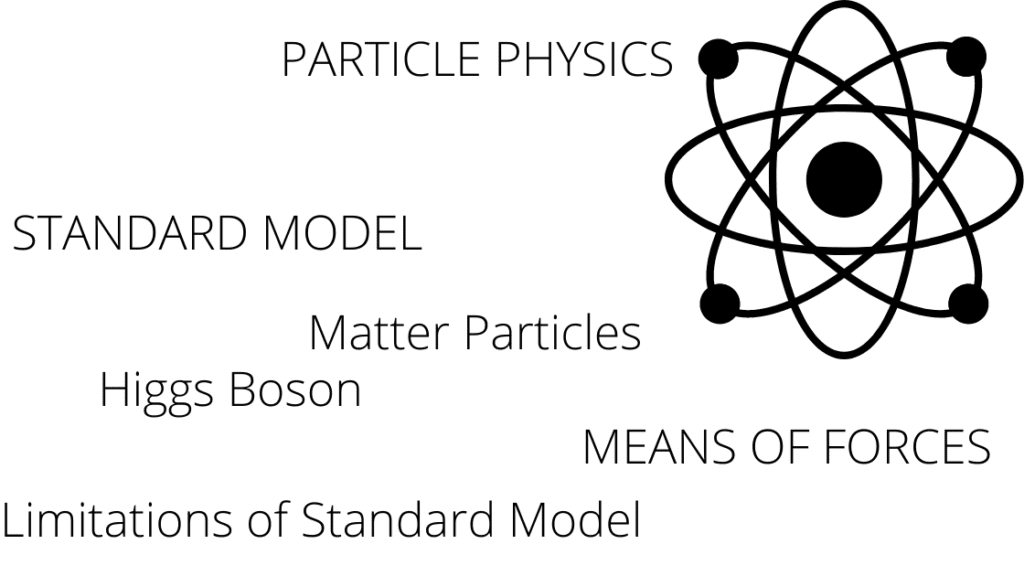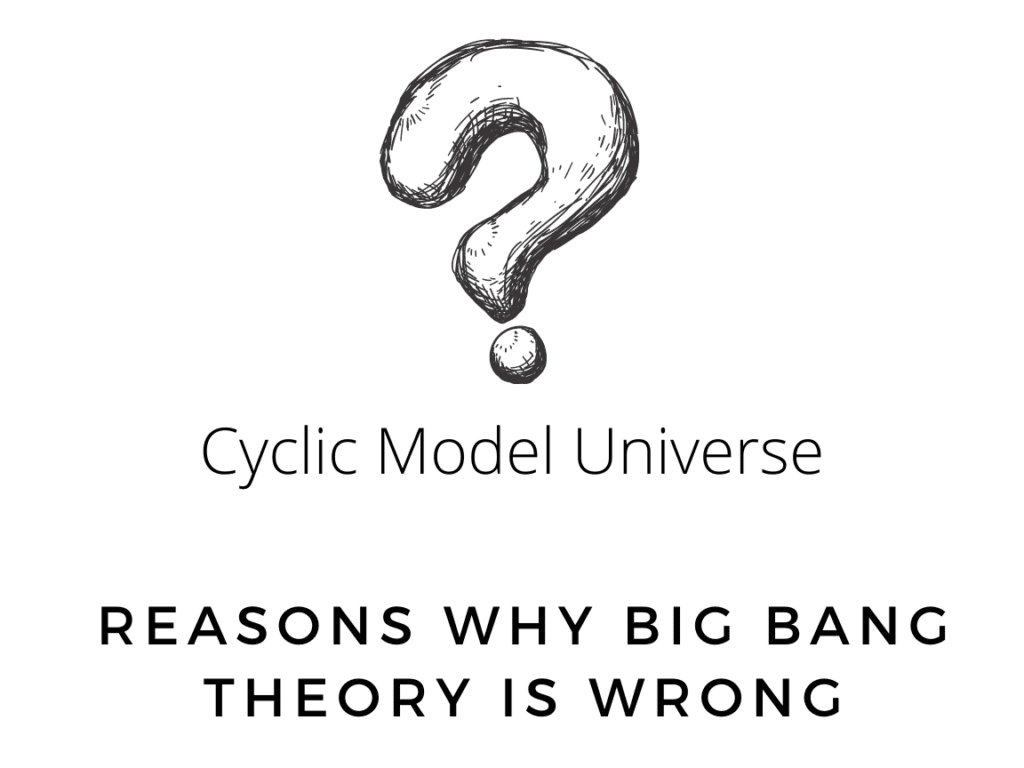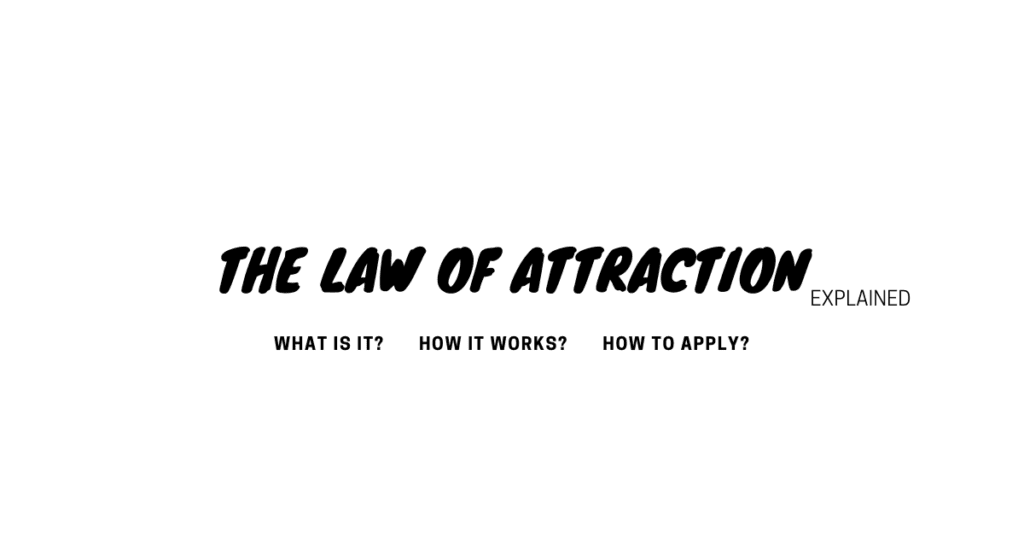# Matter & Energy

Matter & Energy- Matter is the substance that constitutes the observable universe and, together with energy, forms the basis of all objective phenomena## Does Milky way contain Dark Matter?

What is Dark matter? Dark matter is a major component of Cosmology and Astrophysics. In the first case, the cosmological models of galactic evolution are based on this dark matter that does not emit or block light. Since it is assumed that it constitutes 85% of the total matter, the models are based on this …## What is General theory of relativity? Equations & Examples

The General Theory of Relativity was published by Albert Einstein in 1915. The General Theory of Relativity is the accepted name published by Albert Einstein in 1915 for the theory of gravity. The force of gravity is a representation of the local geometry of space-time, according to the General Theory of Relativity. While the modern …## Particle Physics Standard Model

What is the Standard Model of Particle Physics? A theory explaining the relationships between known fundamental interactions between elementary particles that make up all matter is the standard model of particle physics. It is a quantum field theory that is consistent with quantum mechanics and special relativity that was developed between 1970 and 1973. Nearly …## Gravity Waves

WASHINGTON, U.S. and European scientists are teaming up to study one of the universe’s most elusive secrets — mysterious “gravity waves” that result when enormous objects collide in space. What they learn could shed new light on an old concept, that collisions between galaxies or stars create waves of gravity just as explosions produce bursts …## Cyclic Model of Universe – Why Big Bang theory is Wrong

Reasons why Big Bang theory is wrong Cyclic Model of Universe proves Big bang theory wrong. Let’s take a theoretical block of the Cosmos that contains 100 billion light-years of space. In the middle of that giant block of the cosmos, we will put Edwin Hubble and his telescope. When Hubble looks in both directions …## Facts of Universe Cycle/Oscillating Model

Cyclic Universe Theory The Universe functions as a cycle. We can first look at how physics equations actually operate to better understand the Universe Cycle. The three factors that go into the equation are there. Balance- There needs to be balance. Equation should have a balance or equality on both sides. The difference- There must be opposites between the two sides of the equation. That …## The Law Of Attraction Explained with Proven Facts & examples

We have heard of the term ‘Law of Attraction’. Lets understand it from top to bottom. What is the Law of Attraction? The law of attraction (LOA) is the idea that the world makes and gives us what our minds focus on. The law of attraction is always working like the law of gravity and it’s true that it is working right now while you’re reading the article. ‘The law of vibration’ and the …